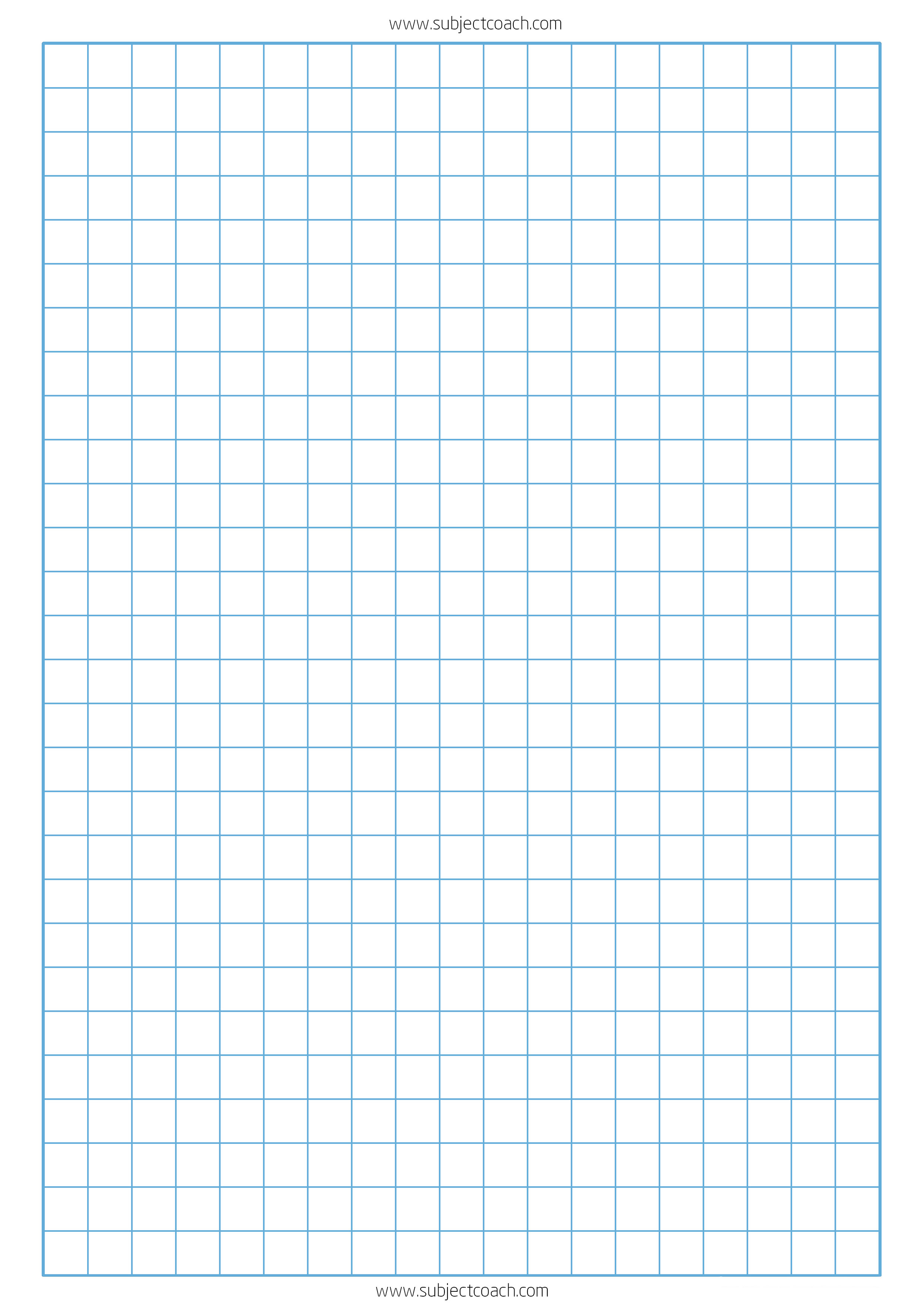# Printable Graph Paper 1cm

Graph paper is one of the most important mathematical tool when you learn graphing, precision drawing etc.

It is a piece of paper that can help you achieve several mathematical skill. Graph paper has several names and comes in different sizes such as graph paper 1cm.

Other than being called a graph paper, it is also called a coordinate paper, squared paper and grid paper as well. It is printed with fine lines forming squares.

The bigger squares have tinier squares and every single square is precisely measured and all of them have the same dimensions. It can be used in several mathematical applications including statistics.

We thought you will like an occasional FREEbie, Here you can find our printable graph paper with 1cm grid that is specially designed to fit an A4 paper.

You can print as many to create your own maths squared paper book, children under your supervision can thus make use of.

Our 1cm grid paper is quick to print and comes in blue lines only and will print grey on a monochrome printer. Use it for maths, drawing, designing, patterns etc.License: You can use this sheet in your school or at home, commercial and non-commercial use is allowed. You cannot modify this document.

## What are the uses of a graph paper?

The graph paper is used in mathematics for different things:

1. To draw empirical data and check the results. A large amount of data can be represented graphically on the graph paper. It is a very useful tool for mathematicians.
2. It is used to draw linear diagrams which are useful for engineers. Mathematical experts use graph paper to draw coordinates and study linearity, similarity and congruence. Linear formulas’ are important mathematical functions and have several uses including physics.
3. It is used by engineers to draw two-dimensional figures like cubes and cylinders. The graph papers are therefore useful for architects who are always drawing 2-dimensional figures to create structures.

### How does a graph paperwork?

When you use a graph paper you shouldn’t worry much about exact measurements for a grid box. It is impossible to draw graphs on a plain sheet of paper, especially graphs that show complicated data. Using a graph paper makes the data clear and one can study the graph very easily. For example, to show a graph for inflation on a plain sheet of paper is confusing and difficult. However, on a graph paper, the rise and fall can be clearly indicated. Similarly, to show architectural designs, the dimensions can easily be shown on a graph paper. You can create complex and symmetric designs using a graph paper.

### Who uses a graph paper?

Graph paper can be used by anyone who has maths as their subject. Even middle school children use graph paper when they start learning statistics or drawing in general. Engineers use graph paper in several different ways. Likewise architects, statisticians, linear programmers, school students and many more people use graph sheets to accomplish some of their respective tasks. These days graph paper is available in various formats online. You can also use digital copies of the graph sheets to create digital diagrams.

Some of our useful material on subjects related to Maths and english: Naplan Practice Test, free typing tutor for kids, free storybooks, and printable maths worksheets for teachers and students to teach and learn faster.

Keep Learning

SubjectCoach Team